# Short Note Prestack migration by split-step DSR

Alexander M. Popovici

mihai@sep.stanford.edu

INTRODUCTION The DSR prestack migration equation, though defined for depth variable velocity, can be used to image media with strong velocity variations using a phase-shift plus interpolation (PSPI) or split-step correction. The split-step method is based on applying a phase-shift correction to the extrapolated wavefield, a correction that attempts to compensate for the lateral velocity variations. I show how to extend DSR prestack migration to lateral velocity media and exemplify the method by applying the new algorithm to the Marmousi dataset.

Split-step prestack migration The depth-variable-velocity prestack migration in offset-midpoint coordinates (Yilmaz, 1979) is formulated as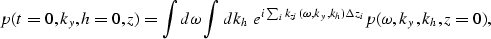(1)
where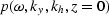is the 3-D Fourier transform of the field p(t,y,h,z=0) recorded at the surface, using Claerbout's (1985) sign convention: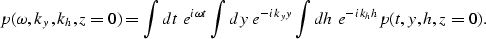The phase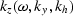is defined in the dispersion relation as(2)
The two integrals inand kh in equation (1) represent the imaging condition for zero-offset and zero time (h=0,t=0).

In parallel, the depth-variable-velocity zero-offset migration (Gazdag, 1978) is formulated as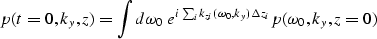(3)
where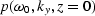is the 2-D Fourier transform of the field p(t,y,z=0). The phase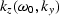is defined in the dispersion relation as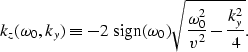(4)

For zero-offset migration, the idea of a split-step correction appeared first in Gazdag and Sguazzero's (1984) PSPI algorithm, but it did not become a standalone technique until Stoffa et al. (1990) reversed the order of the algorithm, permitting the correction to be applied only once. The schematic flow of the two algorithms is shown in Figure, and the split-step correction is represented by the box containing the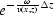term.

gazsplitheight=3.2in.The two Fourier migration algorithms.
a) Phase Shift Plus Interpolation (PSPI).
b) Split-step Fourier Migration.

Gazdag and Sguazzero (1984) implement a technique in the PSPI algorithm to ensure that all the zero dips (corresponding to the case kx=0) are downward continued without distortion. The technique consists of multiplying the wavefieldwith the phase-shift correction factor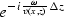prior to the Fourier transformation along the X axis. The downward extrapolation phase incorporates another correction factor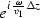where the subscript v1 denotes one of the constant velocities used in the downward extrapolation step. When the velocity varies only in depth v(z), the two terms cancel each other. When the reflectors are flat (kx=0), the extrapolation term becomesand is cancelled by the multiplication with the phase-shift correction factorAs a result, the zero-dip reflections are downward continued with the correct laterally varying phase-shiftThe same result can be obtained via the Stoffa et al. (1990) algorithm, where the phase-correction term is contained in a single term, as follows: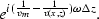The difference is that the downward extrapolation is done with a single velocity and the perturbation slowness phase shift is performed after the downward extrapolation step.

For prestack migration, the two square roots in the phase term represent specifically the extrapolation term for the source and for the receiver. In other words if we downward extrapolate first the source, and second the receiver, we need in each case a different phase-correction term. The phase-correction term includes a laterally varying velocity function, vg(xg,z) for the geophone-field continuation and vs(xs,z) for the source-field continuation. A minor problem arises in the fact that the DSR prestack migration equation (1) is defined in midpoint and offset coordinates, while the velocity is defined in shot and geophone coordinates. We can easily go from one system to another using the transformation relations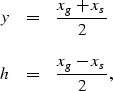(5)
where y and h are the midpoint and offset coordinates and xs and xg are the shot and geophone surface coordinates. Note that the variable h represents half the total distance between the source and geophone.

The split-step correction term becomes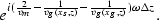In midpoint-offset coordinates the split-step correction term becomes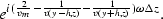In other words, for each midpoint y, we use the velocity located at y-h (the source location) and the velocity located at y+h (the receiver location). A diagram of the prestack migration split-step algorithm is represented in Figure. The last box represents the multiplication with the split-step correction exponential term, where the slowness difference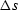is defined as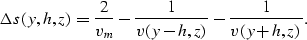DSRsplitheight=3.2in. DSR split-step prestack migration algorithm.

The DSR split-step migration algorithm was applied to the Marmousi data, a well known prestack synthetic dataset generated by the Institut Français du Pétrole. The Marmousi dataset is based on a real geologic model from the Cuanza basin in Angola (Aline Bourgeois et al, 1991). The geological model of the basin consists of a deltaic sediment interval deposited upon a saliferous evaporitic series. The sediments are affected by normal growth faults caused by the salt creep. Under the salt there is a folded carbonate sedimentation series forming a structural hydrocarbon trap. The challenge presented to the exploration geophysicists it to image the hydrocarbon trap. The complex velocity model, with strong lateral velocity variations, is shown in Figurea. Figureb shows the near-offset section with a half-offset h=100 m.

Figurea shows the result of zero-offset split-step migration applied to the near-offset section. The full near offset is 200 meters (h=100 m). Applying a zero-offset algorithm to a non-zero offset section obviously introduces errors in the imaging results, and the target area is poorly imaged. In comparison, the DSR split-step prestack migration algorithm produces an excellent image of the hydrocarbon trap, shown in Figureb.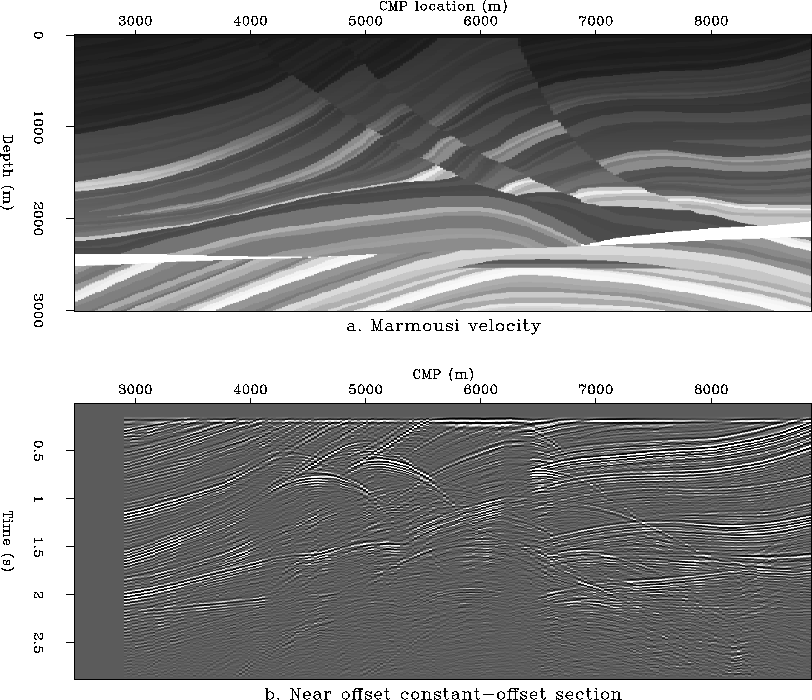marmVelandNO
Figure 1
Marmousi velocity model and near-offset section.
a. Marmousi velocity model.
b. Near-offset section (h=100 m).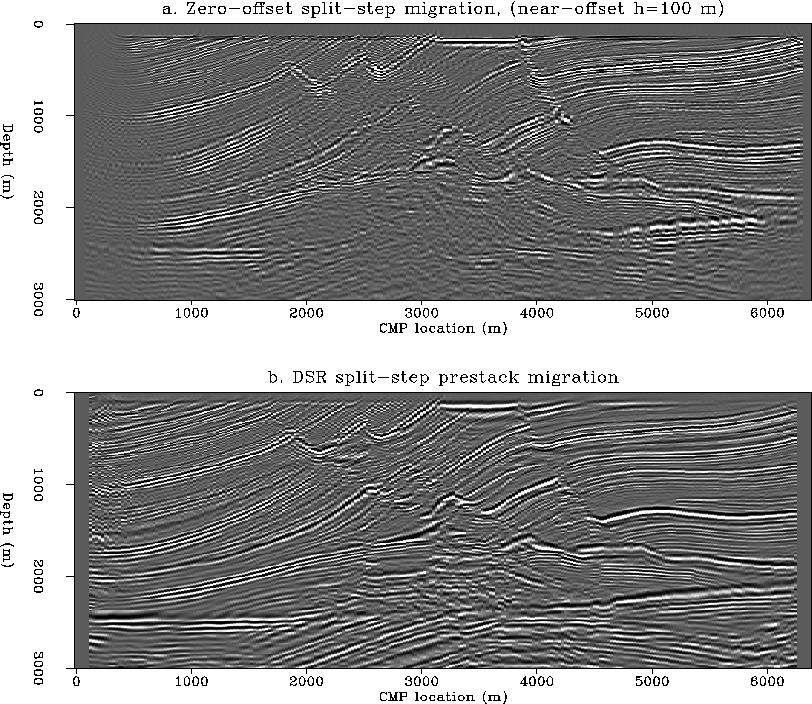ZOandDSRsplit
Figure 2
Zero-offset and prestack migration of the Marmousi.
a. Zero-offset migration of the near-offset section.
b. Prestack migration using DSR with a split-step term.The size of the prestack migration dataset used was 720x256x64 and was increased to 1024x256x64 by zero padding for the fast Fourier transform. The prestack migration took about 20 minutes on a 1024x256x64 dataset. The prestack migration took about 20 minutes on our CM-5 when using the entire frequency spectrum (1024 frequencies). The runtime can be effectively cut by a factor of two by using only half of the total number of frequencies. My implementation also sums implicitly over the offset. By using the artifact reduction techniques described in Popovici (1993) and stationary phase tables, the algorithm can be modified to handle separate offsets.

CONCLUSIONS A very simple split-step modification to DSR prestack migration leads to a powerful migration algorithm that can handle strong lateral velocity variations. The new algorithm produces very good images of the Marmousi dataset and is computationally very efficient on our CM-5.

REFERENCES

• Gazdag, J., 1978, Wave equation migration with the phase shift method: Geophysics, 43, 1342-1351.

• Gazdag, J., and Sguazzero, P., 1984, Migration of seismic data by phase shift plus interpolation: Geophysics, 49, 124-131.

• Popovici, A. M., 1993, Prestack phase-shift migration for separate offsets: SEP-79, 115-127.

• Stoffa, P. L., Fokkema, J. T., de Luna Freire, R. M., and Kessinger, W. P., 1990, Split-step Fourier migration: Geophysics, 55, 410-421.

• Yilmaz, O.,1979, Prestack partial migration: Ph.D. thesis, Stanford University.